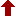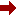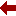#### SLIM21

 Sort Order Chronological Author Title ItemType,Author ItemType,Title Display Format Tabular Publication Bibliography Items / Page 5 10 25

Click the serial number on the left to view the details of the item.
#  AuthorTitleAccn#YearItem Type Claims
1 Landi, Giovanni Linear Algebra and Analytic Geometry for Physical Sciences I09687 2018 eBook
2 Ydri, Badis Lectures on Matrix Field Theory I08731 2017 eBook
3 Liseikin, Vladimir D A Computational Differential Geometry Approach to Grid Generation I08014 2007 eBook
4 Hubbard, John H MacMath 9.2 I04475 1993 eBook
 (page:1 / 1) [#4]

1.TitleLinear Algebra and Analytic Geometry for Physical Sciences
Author(s)Landi, Giovanni;Zampini, Alessandro
PublicationCham, 1. Imprint: Springer 2. Springer International Publishing, 2018.
DescriptionXII, 345 p : online resource
Abstract NoteA self-contained introduction to finite dimensional vector spaces, matrices, systems of linear equations, spectral analysis on euclidean and hermitian spaces, affine euclidean geometry, quadratic forms and conic sections. The mathematical formalism is motivated and introduced by problems from physics, notably mechanics (including celestial) and electro-magnetism, with more than two hundreds examples and solved exercises. Topics include: The group of orthogonal transformations on euclidean spaces, in particular rotations, with Euler angles and angular velocity. The rigid body with its inertia matrix. The unitary group. Lie algebras and exponential map. The Dirac???s bra-ket formalism. Spectral theory for self-adjoint endomorphisms on euclidean and hermitian spaces. The Minkowski spacetime from special relativity and the Maxwell equations. Conic sections with the use of eccentricity and Keplerian motions. An appendix collects basic algebraic notions like group, ring and field; and complex numbers and integers modulo a prime number. The book will be useful to students taking a physics or engineer degree for a basic education as well as for students who wish to be competent in the subject and who may want to pursue a post-graduate qualification
ISBN,Price9783319783611
Keyword(s)1. ALGEBRA 2. APPLIED MATHEMATICS 3. Computer science???Mathematics 4. EBOOK 5. EBOOK - SPRINGER 6. ENGINEERING MATHEMATICS 7. GEOMETRY 8. Linear and Multilinear Algebras, Matrix Theory 9. Math Applications in Computer Science 10. Mathematical and Computational Engineering 11. Mathematical Applications in the Physical Sciences 12. Mathematical Methods in Physics 13. MATHEMATICAL PHYSICS 14. MATRIX THEORY 15. PHYSICS
Item TypeeBook
Circulation Data
 Accession# Call# Status Issued To Return Due On Physical Location I09687 On Shelf

2.TitleLectures on Matrix Field Theory
PublicationCham, 1. Imprint: Springer 2. Springer International Publishing, 2017.
DescriptionXII, 352 p. 8 illus., 6 illus. in color : online resource
Abstract NoteThese lecture notes provide a systematic introduction to matrix models of quantum field theories with non-commutative and fuzzy geometries.?? The book initially focuses on the matrix formulation of non-commutative and fuzzy spaces, followed by a description of the non-perturbative treatment of the corresponding field theories. As an example, the phase structure of non-commutative phi-four theory is treated in great detail, with a separate chapter on the multitrace approach. The last chapter offers a general introduction to non-commutative gauge theories, while two appendices round out the text. Primarily written as a self-study guide for postgraduate students ??? with the aim of pedagogically introducing them to key analytical and numerical tools, as well as useful physical models in applications ??? these lecture notes will also benefit experienced researchers by providing a reference guide to the fundamentals of non-commutative field theory with an emphasis on matrix models and fuzzy geometries
ISBN,Price9783319460031
Keyword(s)1. ALGEBRAIC GEOMETRY 2. Computer science???Mathematics 3. EBOOK 4. EBOOK - SPRINGER 5. Math Applications in Computer Science 6. MATHEMATICAL PHYSICS 7. Quantum Field Theories, String Theory 8. QUANTUM FIELD THEORY 9. QUANTUM PHYSICS 10. STRING THEORY
Item TypeeBook
Circulation Data
 Accession# Call# Status Issued To Return Due On Physical Location I08731 On Shelf

3.TitleA Computational Differential Geometry Approach to Grid Generation
PublicationBerlin, Heidelberg, 1. Imprint: Springer 2. Springer Berlin Heidelberg, 2007.
DescriptionXIV, 294 p. 81 illus., 3 illus. in color : online resource
Abstract NoteThe process of breaking up a physical domain into smaller sub-domains, known as meshing, facilitates the numerical solution of partial differential equations used to simulate physical systems. This monograph gives a detailed treatment of applications of geometric methods to advanced grid technology. It focuses on and describes a comprehensive approach based on the numerical solution of inverted Beltramian and diffusion equations with respect to monitor metrics for generating both structured and unstructured grids in domains and on surfaces. In this second edition the author takes a more detailed and practice-oriented approach towards explaining how to implement the method by: Employing geometric and numerical analyses of monitor metrics as the basis for developing efficient tools for controlling grid properties. Describing new grid generation codes based on finite differences for generating both structured and unstructured surface and domain grids. Providing examples of applications of the codes to the generation of adaptive, field-aligned, and balanced grids, to the solutions of CFD and magnetized plasmas problems. The book addresses both scientists and practitioners in applied mathematics and numerical solution of field problems
ISBN,Price9783540342366
Keyword(s)1. Classical and Continuum Physics 2. Computational Mathematics and Numerical Analysis 3. Computer mathematics 4. Computer science???Mathematics 5. Continuum physics 6. DIFFERENTIAL GEOMETRY 7. EBOOK 8. EBOOK - SPRINGER 9. Math Applications in Computer Science 10. Mathematical Methods in Physics 11. Numerical and Computational Physics, Simulation 12. PHYSICS
Item TypeeBook
Circulation Data
 Accession# Call# Status Issued To Return Due On Physical Location I08014 On Shelf

4.TitleMacMath 9.2 : A Dynamical Systems Software Package for the Macintosh???
Author(s)Hubbard, John H;West, Beverly H
PublicationNew York, NY, 1. Imprint: Springer 2. Springer New York, 1993.
DescriptionVII, 162 p. 1 illus : online resource
Abstract NoteMacMath is a scientific toolkit for the Macintosh computer consisting of twelve graphics programs. It supports mathematical computation and experimentation in dynamical systems, both for differential equations and for iteration. The MacMath package was designed to accompany the textbooks Differential Equations: A Dynamical Systems Approach Part I & II. The text and software was developed for a junior-senior level course in applicable mathematics at Cornell University, in order to take advantage of excellent and easily accessible graphics. MacMath addresses differential equations and iteration such as: analyzer, diffeq, phase plane, diffeq 3D views, numerical methods, periodic differential equations, cascade, 2D iteration, eigenfinder, jacobidraw, fourier, planets. These versatile programs greatly enhance the understanding of the mathematics in these topics. Qualitative analysis of the picture leads to quantitative results and even to new mathematics. This new edition includes the latest version of the Mac Math diskette, 9.2
ISBN,Price9781461383789
Keyword(s)1. COMPLEX SYSTEMS 2. Computer science???Mathematics 3. DYNAMICAL SYSTEMS 4. EBOOK 5. EBOOK - SPRINGER 6. Math Applications in Computer Science 7. STATISTICAL PHYSICS 8. Statistical Physics and Dynamical Systems
Item TypeeBook